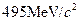Chapter 33, Problem 3PE

Chapter
Section
Textbook Problem

Another component of the strong nuclear force is transmitted by the exchange of virtual K−mesons. Taking K−mesons to have an average mass ofwhat is the approximate range at this component of the strong force?

To determine

The approximate range of this component of the strong force

Explanation

Given info:

Another component of the strong nuclear force is transmitted by the exchange of virtual K- mesons. Taking K-mesons to have an average mass of 495 MeV/c2.

Formula used:

Using the expression,

ΔEΔth4π

Here, Δt is uncertainty time, ΔE is uncertainty energy and h is Plank`s constant.

Expression can also wright,

ΔE=h4πΔt=h4π(d/c)d=hc4πΔE

Calculation:

We have,

h=4.14×1024GeV

Still sussing out bartleby?

Check out a sample textbook solution.

See a sample solution

The Solution to Your Study Problems

Bartleby provides explanations to thousands of textbook problems written by our experts, many with advanced degrees!

Get Started

Find more solutions based on key concepts